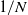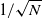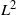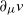Hostname: page-component-594f858ff7-r29tb Total loading time: 0 Render date: 2023-06-07T08:29:58.947Z Has data issue: false Feature Flags: { "corePageComponentGetUserInfoFromSharedSession": false, "coreDisableEcommerce": false, "corePageComponentUseShareaholicInsteadOfAddThis": true, "coreDisableSocialShare": false, "useRatesEcommerce": true } hasContentIssue false

## Abstract

We prove a rate of convergence for the N-particle approximation of a second-order partial differential equation in the space of probability measures, such as the master equation or Bellman equation of the mean-field control problem under common noise. The rate is of order$1/N$ for the pathwise error on the solution v and of order$1/\sqrt{N}$ for the$L^2$ -error on its L-derivative$\partial_\mu v$ . The proof relies on backward stochastic differential equation techniques.

Type
Original Article
Information
Journal of Applied Probability , December 2022 , pp. 992 - 1008

## Access options

Get access to the full version of this content by using one of the access options below. (Log in options will check for institutional or personal access. Content may require purchase if you do not have access.)

## References

Bensoussan, A., Frehse, J. and Yam, P. (2013). Mean Field Games and Mean Field Type Control Theory (Springer Briefs in Mathematics). Springer.CrossRefGoogle Scholar
Bensoussan, A., Frehse, J. and Yam, P. (2015). The master equation in mean-field theory. J. Math. Pures Appl. 103, 14411474.CrossRefGoogle Scholar
Buckdahn, R., Li, J., Peng, S. and Rainer, C. (2017). Mean-field stochastic differential equations and associated PDEs. Ann. Prob. 45, 824878.CrossRefGoogle Scholar
Burzoni, M., Ignazio, V., Reppen, M. and Soner, H. (2020). Viscosity solutions for controlled McKean–Vlasov jump-diffusions. SIAM J. Control Optim. 58, 16761699.CrossRefGoogle Scholar
Cardaliaguet, P., Delarue, F., Lasry, J.-M. and Lions, P.-L. (2019). The Master Equation and the Convergence Problem in Mean-Field Games (Annals of Mathematics Studies 201). Princeton University Press.Google Scholar
Carmona, R. and Delarue, F. (2018). Probabilistic Theory of Mean Field Games, Vol. I, Mean Field FBSDEs, Control, and Games. Springer.Google Scholar
Carmona, R. and Delarue, F. (2018). Probabilistic Theory of Mean Field Games, Vol. II, Mean Field FBSDEs, Control, and Games. Springer.Google Scholar
Chassagneux, J.-F., Crisan, D. and Delarue, F. (2015). A probabilistic approach to classical solutions of the master equation for large population equilibria. Mem. Amer. Math. Soc., to appear.Google Scholar
Cosso, A. and Pham, H. (2019). Zero-sum stochastic differential games of generalized McKean–Vlasov type. J. Math. Pures Appl. 129, 180212.CrossRefGoogle Scholar
Djete, M. F. (2020). Extended mean field control problem: a propagation of chaos result. Electron. J. Prob., to appear. Available at arXiv:2006.12996.Google Scholar
Djete, M. F., Possamaï, D. and Tan, X. (2019). McKean–Vlasov optimal control: the dynamic programming principle. Ann. Prob., to appear. Available at arXiv:1907.08860.Google Scholar
Fournier, N. and Guillin, A. (2015). On the rate of convergence in the Wasserstein distance of the empirical measure. Prob. Theory Related Fields 162, 707738.CrossRefGoogle Scholar
Gangbo, W. and Swiech, A. (2015). Existence of a solution to an equation arising from the theory of mean-field games. J. Differential Equations 259, 65736643.CrossRefGoogle Scholar
Gangbo, W., Mayorga, S. and Swiech, A. (2021). Finite dimensional approximations of Hamilton–Jacobi–Bellman equations in spaces of probability measures. SIAM J. Math. Anal. 53, 13201356.CrossRefGoogle Scholar
Germain, M., Laurière, M., Pham, H. and Warin, X. (2021). DeepSets and derivative networks for solving symmetric PDEs. Available at arXiv:2103.00838.Google Scholar
Lacker, D. (2017). Limit theory for controlled McKean–Vlasov dynamics. SIAM J. Control Optim. 55, 16411672.CrossRefGoogle Scholar
Laurière, M. and Tangpi, L. (2019). Backward propagation of chaos. Electron. J. Prob., to appear. Available at arXiv:1911.06835.Google Scholar
Laurière, M. and Tangpi, L. (2020). Convergence of large population games to mean field games with interaction through the controls. SIAM J. Math. Anal., to appear. Available at arXiv:2004.08351.Google Scholar
Pardoux, E. and Peng, S. (1990). Adapted solution of a backward stochastic differential equation. Systems Control Lett. 14, 5561.CrossRefGoogle Scholar
Pham, H. and Wei, X. (2017). Dynamic programming for optimal control of stochastic McKean–Vlasov dynamics. SIAM J. Control Optim. 55, 10691101.CrossRefGoogle Scholar
Pham, H. and Wei, X. (2018). Bellman equation and viscosity solutions for mean-field stochastic control problem. ESAIM Control Optim. Calc. Var. 24, 437461.CrossRefGoogle Scholar
Saporito, Y. F. and Zhang, J. (2019). Stochastic control with delayed information and related nonlinear master equation. SIAM J. Control Optim. 57, 693717.CrossRefGoogle Scholar
Wu, C. and Zhang, J. (2020). Viscosity solutions to parabolic master equations and McKean–Vlasov SDEs with closed-loop controls. Ann. Appl. Prob. 30, 936986.CrossRefGoogle Scholar
Zhang, J. (2017). Backward Stochastic Differential Equations: From Linear to Fully Nonlinear Theory (Probability Theory and Stochastic Modelling 86). Springer.CrossRefGoogle Scholar